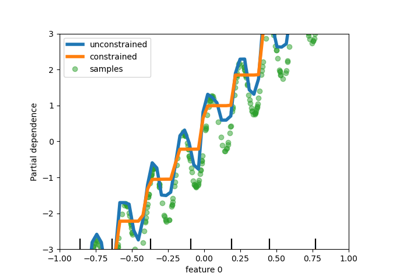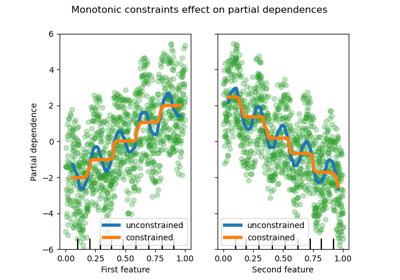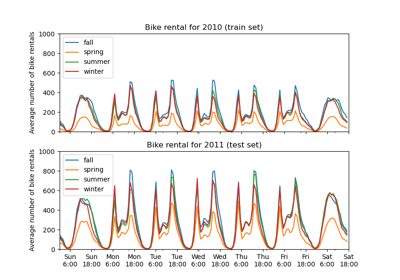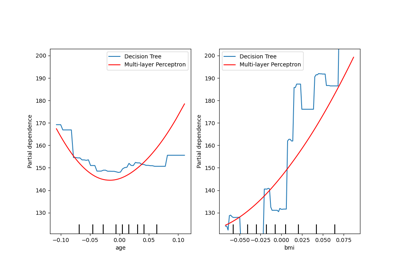# sklearn.inspection.plot_partial_dependence¶

sklearn.inspection.plot_partial_dependence(estimator, X, features, *, feature_names=None, target=None, response_method='auto', n_cols=3, grid_resolution=100, percentiles=0.05, 0.95, method='auto', n_jobs=None, verbose=0, line_kw=None, contour_kw=None, ax=None, kind='average', subsample=1000)[source]

Partial dependence (PD) and individual conditional expectation (ICE) plots.

Partial dependence plots, individual conditional expectation plots or an overlay of both of them can be plotted by setting the kind parameter. The len(features) plots are arranged in a grid with n_cols columns. Two-way partial dependence plots are plotted as contour plots. The deciles of the feature values will be shown with tick marks on the x-axes for one-way plots, and on both axes for two-way plots.

Read more in the User Guide.

Note

plot_partial_dependence does not support using the same axes with multiple calls. To plot the the partial dependence for multiple estimators, please pass the axes created by the first call to the second call:

>>> from sklearn.inspection import plot_partial_dependence
>>> from sklearn.datasets import make_friedman1
>>> from sklearn.linear_model import LinearRegression
>>> from sklearn.ensemble import RandomForestRegressor
>>> X, y = make_friedman1()
>>> est1 = LinearRegression().fit(X, y)
>>> est2 = RandomForestRegressor().fit(X, y)
>>> disp1 = plot_partial_dependence(est1, X,
...                                 [1, 2])
>>> disp2 = plot_partial_dependence(est2, X, [1, 2],
...                                 ax=disp1.axes_)


Warning

For GradientBoostingClassifier and GradientBoostingRegressor, the 'recursion' method (used by default) will not account for the init predictor of the boosting process. In practice, this will produce the same values as 'brute' up to a constant offset in the target response, provided that init is a constant estimator (which is the default). However, if init is not a constant estimator, the partial dependence values are incorrect for 'recursion' because the offset will be sample-dependent. It is preferable to use the 'brute' method. Note that this only applies to GradientBoostingClassifier and GradientBoostingRegressor, not to HistGradientBoostingClassifier and HistGradientBoostingRegressor.

Parameters
estimatorBaseEstimator

A fitted estimator object implementing predict, predict_proba, or decision_function. Multioutput-multiclass classifiers are not supported.

X{array-like or dataframe} of shape (n_samples, n_features)

X is used to generate a grid of values for the target features (where the partial dependence will be evaluated), and also to generate values for the complement features when the method is 'brute'.

featureslist of {int, str, pair of int, pair of str}

The target features for which to create the PDPs. If features[i] is an integer or a string, a one-way PDP is created; if features[i] is a tuple, a two-way PDP is created (only supported with kind='average'). Each tuple must be of size 2. if any entry is a string, then it must be in feature_names.

feature_namesarray-like of shape (n_features,), dtype=str, default=None

Name of each feature; feature_names[i] holds the name of the feature with index i. By default, the name of the feature corresponds to their numerical index for NumPy array and their column name for pandas dataframe.

targetint, default=None
• In a multiclass setting, specifies the class for which the PDPs should be computed. Note that for binary classification, the positive class (index 1) is always used.

• In a multioutput setting, specifies the task for which the PDPs should be computed.

Ignored in binary classification or classical regression settings.

response_method{‘auto’, ‘predict_proba’, ‘decision_function’}, default=’auto’

Specifies whether to use predict_proba or decision_function as the target response. For regressors this parameter is ignored and the response is always the output of predict. By default, predict_proba is tried first and we revert to decision_function if it doesn’t exist. If method is 'recursion', the response is always the output of decision_function.

n_colsint, default=3

The maximum number of columns in the grid plot. Only active when ax is a single axis or None.

grid_resolutionint, default=100

The number of equally spaced points on the axes of the plots, for each target feature.

percentilestuple of float, default=(0.05, 0.95)

The lower and upper percentile used to create the extreme values for the PDP axes. Must be in [0, 1].

methodstr, default=’auto’

The method used to calculate the averaged predictions:

Please see this note for differences between the 'brute' and 'recursion' method.

n_jobsint, default=None

The number of CPUs to use to compute the partial dependences. None means 1 unless in a joblib.parallel_backend context. -1 means using all processors. See Glossary for more details.

verboseint, default=0

Verbose output during PD computations.

line_kwdict, default=None

Dict with keywords passed to the matplotlib.pyplot.plot call. For one-way partial dependence plots.

contour_kwdict, default=None

Dict with keywords passed to the matplotlib.pyplot.contourf call. For two-way partial dependence plots.

axMatplotlib axes or array-like of Matplotlib axes, default=None
• If a single axis is passed in, it is treated as a bounding axes and a grid of partial dependence plots will be drawn within these bounds. The n_cols parameter controls the number of columns in the grid.

• If an array-like of axes are passed in, the partial dependence plots will be drawn directly into these axes.

• If None, a figure and a bounding axes is created and treated as the single axes case.

New in version 0.22.

kind{‘average’, ‘individual’, ‘both’}, default=’average’

Whether to plot the partial dependence averaged across all the samples in the dataset or one line per sample or both.

• kind='average' results in the traditional PD plot;

• kind='individual' results in the ICE plot.

Note that the fast method='recursion' option is only available for kind='average'. Plotting individual dependencies requires using the slower method='brute' option.

New in version 0.24.

subsamplefloat, int or None, default=1000

Sampling for ICE curves when kind is ‘individual’ or ‘both’. If float, should be between 0.0 and 1.0 and represent the proportion of the dataset to be used to plot ICE curves. If int, represents the absolute number samples to use.

Note that the full dataset is still used to calculate averaged partial dependence when kind='both'.

New in version 0.24.

Returns
display: PartialDependenceDisplay

sklearn.inspection.partial_dependence

Return raw partial dependence values

Examples

>>> from sklearn.datasets import make_friedman1

## Examples using sklearn.inspection.plot_partial_dependence¶